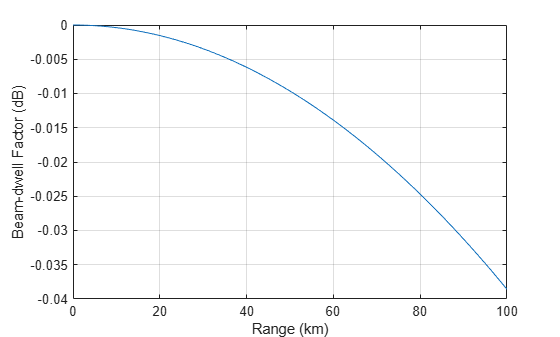# beamdwellfactor

Range-dependent loss for rapidly scanning beam

## Syntax

``fbd = beamdwellfactor(r,hpbw,scanrate)``

## Description

example

````fbd = beamdwellfactor(r,hpbw,scanrate)` calculates the range-dependent beam-dwell factor `fbd` for an antenna at the specified range `r`, half-power beamwidth `hpbw`, and scan rate `scanrate`. The `beamdwellfactor` function assumes that the transmitter and receiver antennas have equal beamwidth and an ideal Gaussian antenna pattern with no side lobes.```

## Examples

collapse all

Calculate the beam-dwell factor for a surveillance radar at 100 linearly-spaced ranges in the interval `[0,100000]` meters. Specify the beamwidth as `1` degree and the scan rate as `120` degrees per second.

```r = linspace(0,100000); hpbw = 1; scanrate = 120; fbd = beamdwellfactor(r,hpbw,scanrate);```

Plot the beam-dwell factor as a function of range. Before plotting, convert the range from meters to kilometers.

```plot(r*0.001,fbd) grid on xlabel('Range (km)') ylabel('Beam-dwell Factor (dB)')```## Input Arguments

collapse all

Range in meters, specified as a scalar or vector.

Data Types: `double`

Half-power beamwidth of the antenna in degrees, specified as a scalar or vector. If `hpbw` is a vector, then `scanrate` must be a scalar or a vector of the same size.

Data Types: `double`

Scan rate of the antenna in degrees per second. If `scanrate` is a vector, then `hpbw` must be a scalar or a vector of the same size.

Data Types: `double`

## Output Arguments

collapse all

Range-dependent beam-dwell factor in dB, returned as a j-by-k matrix such that j is the size of `r` and k is the size of `hpbw` or `scanrate`, whichever is larger.

The rows of `fbd` correspond to the ranges in `r`. The columns depend on the sizes of `hpbw` and `scanrate`.

• If `hpbw` is a vector and `scanrate` is a scalar, then the columns of `fbd` correspond to the half-power beamwidths in `hpbw`.

• If `hpbw` is a scalar and `scanrate` is a vector, then the columns of `fbd` correspond to the scan rates in `scanrate`.

• If `hpbw` and `scanrate` are both vectors, then the columns of `fbd` correspond to both the half-power beamwidths in `hpbw` and the scan rates in `scanrate`.

Data Types: `double`

collapse all

### Beam-Dwell Factor

The beam-dwell factor accounts for the misalignment between transmitter and receiver beam axes when a scanning system has a high scan rate and long-range targets.

The equation for the beam-dwell factor, Fbd, is

`${F}_{bd}=L\underset{-\pi }{\overset{\pi }{\int }}{f}^{2}\left(\theta \right){f}^{2}\left(\theta -\delta \right)d\theta$`

where the terms in the equation are:

• L — Normalizing factor that brings Fbd to unity for = 0

• = td / t0 — Fractional beamwidth scanned during the delay, where:

• td = 2R / c — Time delay for a target, where R is the range and c is the wave propagation speed

• t0 = θ3 / ωs — The time the system takes to continuously scan through one beamwidth, where θ3 is the half-power beamwidth and ωs is the scan rate

• f(θ) — Antenna pattern

The `beamdwellfactor` function assumes an ideal Gaussian antenna pattern with no side lobes. The equation for the ideal Gaussian antenna pattern with no side lobes, f(θ), is:

`$f\left(\theta \right)=\mathrm{exp}\left[-2\left(\mathrm{ln}2\right)\frac{{\theta }^{2}}{{\theta }_{3}^{2}}\right]$`

 Barton, David Knox. "Beam-Dwell Factor Fbd." In Radar Equations for Modern Radar, 362. Artech House Radar Series. Boston, Mass: Artech House, 2013.

 Barton, David Knox. "Antenna Patterns." In Radar Equations for Modern Radar, 147. Artech House Radar Series. Boston, Mass: Artech House, 2013.